Calculated fields are part of Advanced forms, and as such, are included only with the multi-license levels of service.

## Overview

Adobe Sign provides the ability to dynamically update the fields in a document (agreement) based on the data entered by the signer when the agreement is signed. This can be done using Calculated Fields. You can use calculated fields to set up rules within a document (or a document template) before sending it out for signature. These rules get processed when the signer interacts with the document and dynamically update the document based on the fields/values.

Calculated fields can be defined within documents using any of the following mechanisms:

## Calculated Fields Use Cases

Calculated fields can be used for any scenarios where the document needs to be dynamically updated at time of signing. Below are a few sample use cases where calculated fields can be used. These use cases are for illustration purpose only.

1. Setting up an Order Form—Calculated fields can be used to set up an order form in which the signer has the option to select from a list of available items and specify a quantity. Selecting an item from the list automatically updates the form with the price for the selected item. The quantity selected by the signer also results in the total cost of the item being calculated automatically. The form can also incorporate additional calculations for determining total tax due on the order or calculating a volume discount based on the business rules for offering discounts to customers.

2. Automatically populating contract term expiration date—For agreements that allow customers to choose the length of term of the contract at time of signing (6 months, 12 months, 24 months, etc.), a calculated field can be used to automatically populate the end date for the contract based on the selection made by the customer at time of signing the contract.

3. Sign up forms with multiple choices—A calculated field can be used to set up sign-up forms (such as membership forms) where the person signing the form can choose from a set of available options. The choices made by the signer can drive other dynamic aspects of forms, such as the terms the signer is agreeing to, the duration of the contract, and also the total cost.

## Calculated Fields Basics

Any form field, checkbox field or radio button field in an Adobe Sign form can be marked as a calculated field. At time of signing, the signer cannot directly enter a value into a field designated as a calculated field, the value of the field gets automatically calculated based on the rules defined in the form.

A calculated field definition consists of two parts: the expression and the format

 Expression Required Defines the formula/rule on how the value of the designated field gets calculated. Adobe Sign evaluates the expression at time of signing and the resulting value is displayed in the field. Format Optional The resulting value of a calculated field can also be formatted as a date, number, or currency by specifying a format.

Calculated fields can be defined using Adobe Sign text tags, using the Adobe Sign web application, or using PDF form fields. When using the Adobe Sign text tags or PDF form fields to define calculated fields, the expression for the calculation is defined using a directive called “calc”. Formatting of the calculated field can be specified using a directive called “format”.

Example

{{someCalculatedField_es_:signer1:calc(someExpn):format(formatType, someFormat)}}

This example shows text tags being used to define a field within a document called “someCalculatedField”. This field is assigned to the recipient identified as signer1. The value of this field is calculated based on the formula specified in “someExpn”. The type of the resulting value is specified by the parameter “formatType” and formatted based on the format defined as “someFormat”.

The Adobe Sign web application’s authoring environment provides a fully functional expression builder for defining formulas for calculated fields. See Using Expression Builder For Specifying Calculated Fields for more details on building expressions.

## Calculated Field Expressions

The expression defines the formula/rule for determining the value of the calculated field. An expression can consist of one or more of the following elements:

 Literal Specifies an actual value that gets used as part of the expression. Expressions can be string values, numbers, or dates. For example: {{helloField_es_:signer1:calc(“Hello”)}} – This literal sets the value of the field called “helloField” to Hello. {{numField_es_:signer1:calc(10)}} – This literal sets the value of the field called “numField”  to 10. {{date_es_:isdate(format="mmm d, yyyy")}} - This literal includes a comma, which is a special delimiter. For the comma to be part of the formatted string, the expression must be cast as a literal value. {{date_es_:isdate(format='d "day of" mmmm, yyyy')}} - It is possible to insert a literal string of characters into a date calculation.  The literal string must be enclosed in either single or double quotes.  The above calculation would produce a string like:  12 day of December, 2012 Strings that need to be included in an expression must be surrounded in quotes (either double (") or single (')). If the resulting value needs to include a quote (either a single quote or a double quote), this can be achieved by using a back slash (\) to close the quote. Important Note: For all examples going forward, only the expression will be shown, the complete syntax for the text tag will not be shown. Operators Operators are used to perform one or more operations as part of the expression. For example: • 6 + 4 – This operator results in the value of 10 being populated in the calculated field. • 5/22/2016 - 3 – This operator results in the value of 5/19/2016 being populated in the calculated field. See Supported Operators for a list of the operators supported within expressions. Fields Existing fields within a document can be used to define an expression by referring to the field by its name. The fields can be Adobe Sign fields (such as: Signature, Date Field, Signer Name, Signer Company, etc.) or fields defined within the document or other calculated fields. For example: • price * quantity – This expression references two other fields within the document called “price” and “quantity”. The result of the multiplication of the values of these two fields will be populated in the Calculated Field. • signerName & “employee of “ & signerCompany – This expression references two fields within the document “signerName” and “signerCompany” which get pre-populated by Adobe Sign if available and concatenates them together. If the signer’s name is “Casey Jones” and the signer’s company is “Acme Corp”, this expression will result in a value of “Casey Jones employee of Acme Corp” being populated in the calculated field. If a field name contains a space, the field name must be placed within a square bracket ([]) to be able to reference it in an expression. For example [field name with spaces] will reference a field in the document called “field name with spaces”. Functions Expressions can also include one or more functions from the Adobe Sign list of supported functions. The specified functions get evaluated during the agreement signing process and the resulting value gets displayed in the calculated field. For example: • dateAdd(d, signedDate, 3)– This expression uses the “dateAdd" function and specifies adding 3 days to the date on which this agreement gets signed. So if the document gets signed on 5/22/2016, the resulting value of the expression will be 5/25/2016. Negative numbers can be used to effectively subtract from the date. See Supported Functions for a list of functions supported within expressions.

#### Supported Operators

The following operators are supported in calculated fields.

 + Add two numbers together. - Subtracts two numbers. / Divides two numbers. * Multiplies two numbers together. & Concatenate two strings together. = Equals. Compares two values and returns true if the two values are equal and false if they are not. != Not Equals. Compares two values and returns true if the two values are not equal and false if they are. < Less Than. Compares two values and returns Boolean (true or false) if the first value is less than the second value. >= Greater than or Equals. Compares two values and returns Boolean (true or false) if the first value is greater than or equal to the second value. <= Less Than or Equals. Compares two values and returns Boolean (true or false) if the first value is less than or equal to the second value. > Greater than. Compares two values and returns Boolean (true or false) if the first value is greater than the second value. % Modulo Operator. Returns the remainder of the division between two numbers. ^ Exponent Operator. Returns the exponential value of the specified numbers.

#### Supported Functions

The following functions are supported when specifying expressions within a calculated field.

 Function Category Description abs(number1) Math Returns the absolute value of the number “number1”. roundUp(number1) Math Round up the number “number1”. roundDown(number1) Math Round down the number “number1”. round(number1) Math Round the number “number1” to nearest integer. min(number1,number2) Math Returns the smaller of the two numbers “number1” and “number2”. max(number1,number2) Math Returns the larger of the two numbers “number1” and “number2”. datePart(part, date) Date Returns the part of the date specified by the first argument as an integer. For example: datePart(m, 5/22/2016) will return the 5, the month part of the date. See Accepted Values for Portions of Dates below for information on the acceptable values for part attribute. dateAdd(part, date, addition) Date Adds the specified number of parts (e.g., "d" for day, "m" for month, or "y" for year) to a date to calculate a future date. You may use numbers or field names. For example: dateAdd("m", "Jan 1, 2016", 3) would return a date of Apr 1, 2016. In this example, the formula adds 3 months to the date specified. Negative numbers can be used to effectively subtract from the date. dateDiff(part, date1, date2) Date Returns the number of parts (e.g., "d" for days, "h" for hours, or "n" for minutes) between two dates. You may use numbers or field names. For example: dateDiff("d", "Jan 1, 2016", "Jan 2, 2016") would return a value of 1 to represent 1 day between January 1st and January 2nd. daysIn(part, date) Date Returns the number of days contained in the part (e.g., "d" for day, "m" for month, or "y" for year) for the date specified. You may use numbers or field names. For example: daysIn("m", "Feb 1, 2016”) returns a value 29 for the days in February 2016 and daysIn("y", "02/01/2015") returns 365 for the days in 2015. date(string) Date Convert a string value such as "1/1/2016" into a date object that can be used in other functions. now() Date Return the date and time when the formula was evaluated, most often when the eSign page is loaded. if(logical_test, value_if_true, value_if_false) Logic Evaluates "logical_test". The “logical_test” can be another expression. If it is TRUE, it returns the value specified in the "value_if_true". If it is FALSE it returns the value of "value_if_false". Both the “value_if_true” and “value_if_false” attributes can also be expressions. For example: if (1>0, "Correct", "Incorrect") would return "Correct". and(logical1, logical2, ...) Logic Returns true only if all the logical arguments evaluate to TRUE. Otherwise it returns false. For example: and (1>0, 2>1) would return "true" and (1>0, 2=1) would return "false". or(logical1, logical2, ...) Logic Returns true if any of the logical arguments (logical1, logical2,...) evaluate to TRUE. If all the logical arguments are evaluated to FALSE, it returns false. For example: or (1>0, 2>1) will return "true". or (1>0, 2=1) will return "false". not(logical) Logic Returns the opposite value of "logical". So if "logical" is evaluated as FALSE, it returns true. If it is evaluated as TRUE, it returns false. For example: not (1>0) would return "false". lookup(sourceField,("value1=return1,value2=return2", "default_value") Logic Evaluates a source field "return value" (used in dropboxes and radio buttons) to populate a default value into the calculated field, based on a list of possible values. For example: lookup(stateField, “CA=California,UT=Utah,MA=Massachusetts,WA=Washington”,”None”) Would return California if stateField was CA, or Utah is stateField was UT, etc.  If no match is found, it would return None.

#### Accepted Values for Portions of Dates

The following values can be used in date related functions when specifying the portion of the date on which the function should operate.

 Part Portion of the date to which the function will apply y Year q Quarter m Month d Day h Hour n Minute s Second

## Calculated Fields Formatting

In addition to specifying the expression for a calculated field the output value can also be formatted. The output can be formatted as text (the default) or formatted as a number or a date.

When used with text tags, the format directive takes two arguments: FormatType and Format.

 FormatType Specifies the type of value the calculated field represents. Accepted values for this parameter are: number and date. Format Specifies the structure of the output value to be placed in the calculated field.

#### Formatting Calculated Fields as Numbers

The following characters can be used to specify the formatting of an output in the form of a number

 Format Character Description _ (Underscore.) Represents any digit placeholder. 9 Represents any digit placeholder. . Location of a mandatory decimal point (or locale-appropriate symbol). 0 Located to the left or right of a mandatory decimal point. Pads with zeros. ( ) If number is less than zero, puts parentheses around the mask. + Places a plus sign before positive number; a minus sign before negative number. - Places a space before positive number; a minus sign before negative number. , Separates every third decimal place with a comma (or locale-appropriate separator character). \$ Places a dollar sign (or locale-appropriate symbol) before formatted number. When specified it must be the first character for the format string. % Converts the number value in the field into a percentage. Multiplies it by 100 and puts the % sign at the end. When specified it must be the last character of the format string.

#### Formatting Calculated Fields as Dates

The following characters can be used to specify the formatting of an output in the form of a date.

 Format Description Example s The seconds of the minute between 0-59. "0" to "59" ss The seconds of the minute with leading zero if required. "00" to "59" n The minute of the hour between 0-59. "0" or "59" nn The minute of the hour with leading zero if required. "00" or "59" h The hour of the day between 1-12. "1" to "12" hh The hour of the day with leading zero if required. "01" to "12" H The hour of the day between 0-23. "0" to "23" HH The hour of the day with leading zero if required. "00" to "23" d The day of the month between 1 and 31. "1" to "31" dd The day of the month with leading zero if required. "01" to "31" ddd Abbreviated day name. "Mon" to "Sun" dddd The full day name. "Monday" to "Sunday" m The month of the year between 1-12. "1" to "12" mm The month of the year with leading zero if required. "01" to "12" mmm Abbreviated month name. "Jan" to "Dec" mmmm The full month name. "January" to "December" yy The year as a two-digit number. "99" or "15" yyyy The full four digit year. "1999" or "2015" t Displays the first character of the A.M./P.M. designator. "A" or "P" tt Displays the A.M./P.M. designator. "AM" or "PM"

#### Formatting Examples

The following examples show how to format date and number calculated fields.

• A Calculated Field formatted as a date:

{{myField_es_:calc(otherDateField + 3):format(date, "mmm dd, yyyy")}}

The output will be something like “May 22, 2016”.

• A Calculated Field formatted as a number.

{{myTax_es_:calc(total * taxrate):format(number, "\$,.00") }}

The output will be something like “\$1,987.65”.

#### Using Expression Builder to Specify Calculated Fields

Calculated fields can be specified through the Adobe Sign authoring environment. The steps below will guide you through the steps of defining a calculated field once you are in the authoring environment.

1. Drag-and-drop a field in the document. Double click on the field that you just added to bring up the field properties dialog. Give the field an appropriate name. Change the Value Type attribute using the drop-down list to be of type Calculated Value. This indicates that the field value will be determined based on a calculation at time of signing.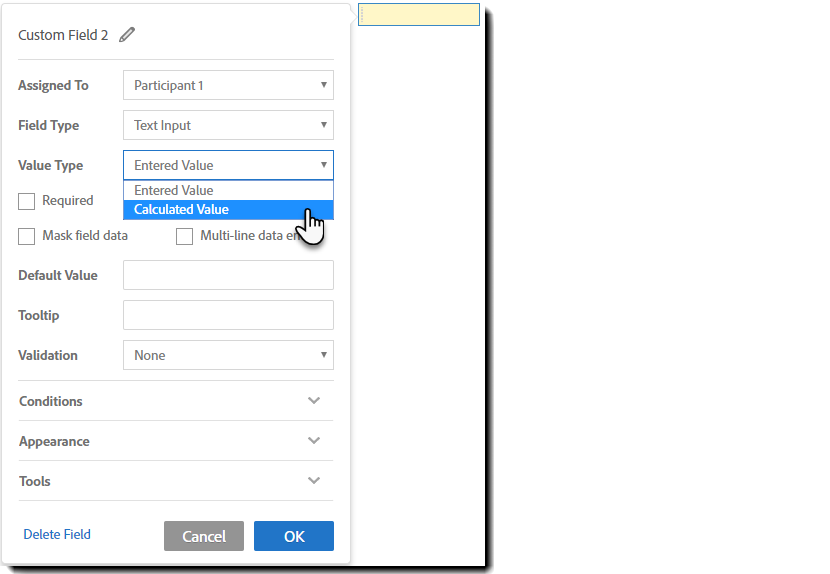2. Type the calculation expression in the text field called Formula or click on the function button ( fx ) to bring up the Expression Builder.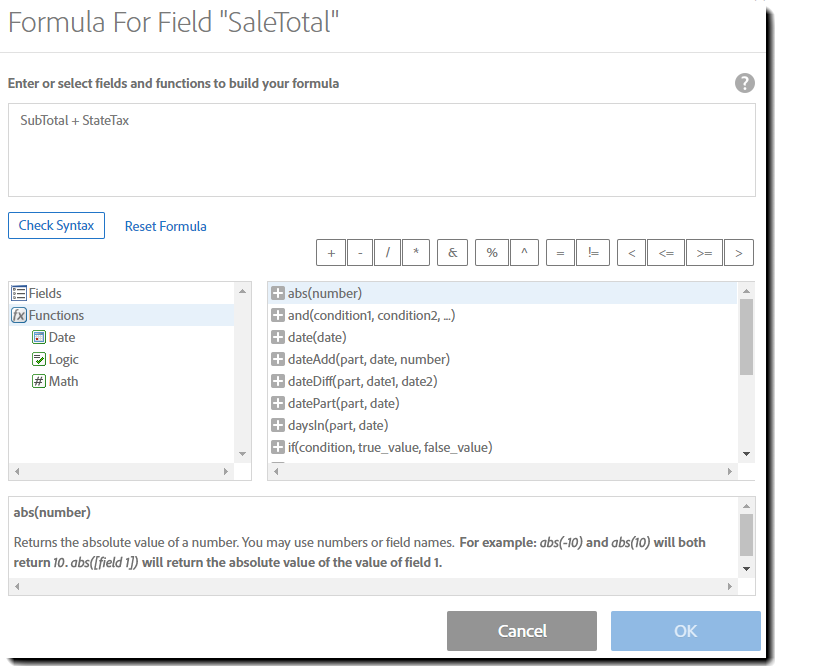3. Use the Expression Builder to define the expression for the calculated field (see Figure below). The Expression Area at the top shows the formula that will be used to calculate the value of the field. You can type the formula into the Expression Area or use the authoring environment to construct the formula. The Expression Builder provides a list of all the available Functions, a list of existing form Fields in the document, and a list of supported Operators to assist in the construction of expressions. You can add a Field or a Function to a formula by double clicking on the item in the List Area in the Expression Builder. You can also add Operators to the expression by clicking on the specific operator in the Operators List.

4. Once you have finished defining the expression, you can check the syntax of the expression by clicking the Check Syntax button then saving the changes.

5. Set the type of the calculated fields using the Display As drop-down list.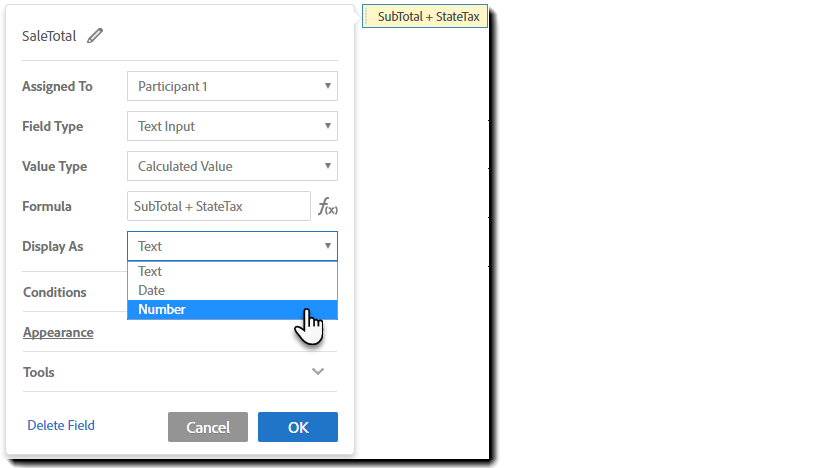6. If your Display As value is Date or Number, set the field value Date/Number Format using the drop-down list. You can also select Custom formatting to define a custom formatting for the field.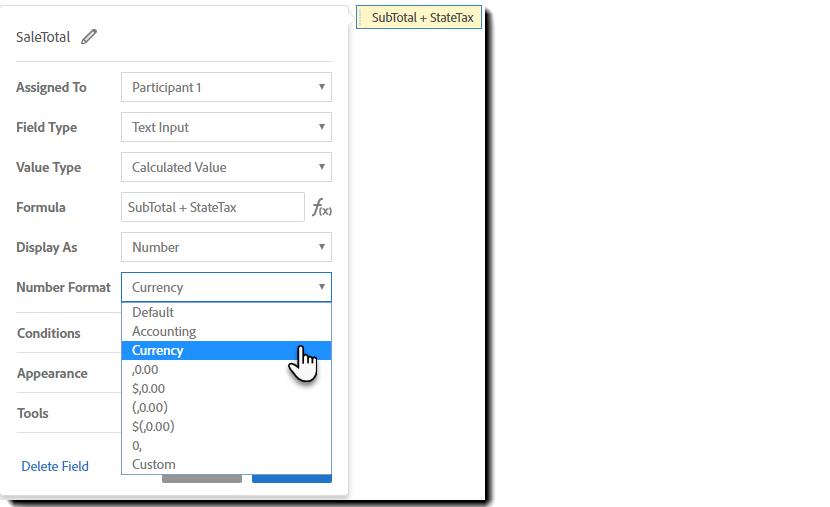## Calculated Fields with a Currency format

The Currency format for a calculated field inserts a currency symbol before the calculated value. (The calculation is otherwise simple math as dictated by the formula.)

Which symbol is inserted is based on the below rules:

• If the source fields contain a Currency validation for the US, the displayed symbol is the US Dollar: \$
• If the source fields contain a Currency validation for the UK, the displayed symbol is the Pound sterling: £

For the Currency formatting to work, the calculated field must be configured with:

• the Display As value set to Number
• the Number Format value set to Currency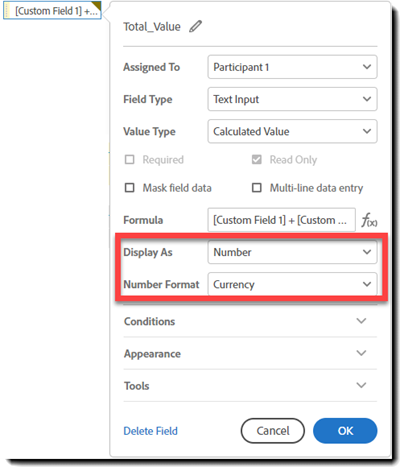The source fields that are used in the calculation can be either Number or Currency validated: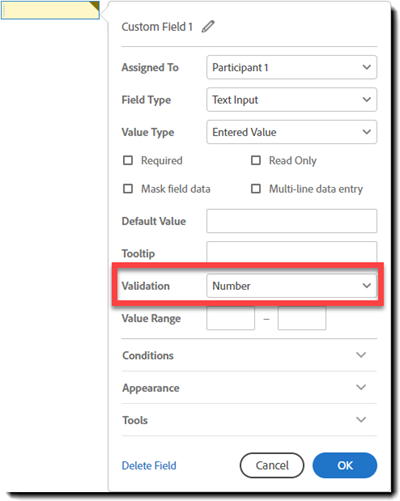Number validated source field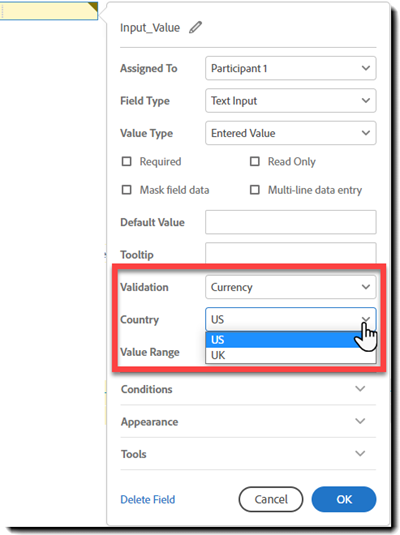Currency validated source field

Увага!

When calculating the value in a Currency formatted field, Adobe Sign is not accounting for any type of currency exchange (Forex).

• The calculated field is performing only the math of the calculation
• The format of the field (Currency) is only inserting the currency symbol

Any form that needs to use a currency symbol other than the Dollar (\$) or Pound (£) must either add the symbol to the document file, or add a read-only field with the currency symbol set as the default value.

When using the field option, it is recommended that the currency symbol field be:

• Assigned To: Sender (Prefill)
• Default Value set to the currency symbol (the Euro is used in the below example)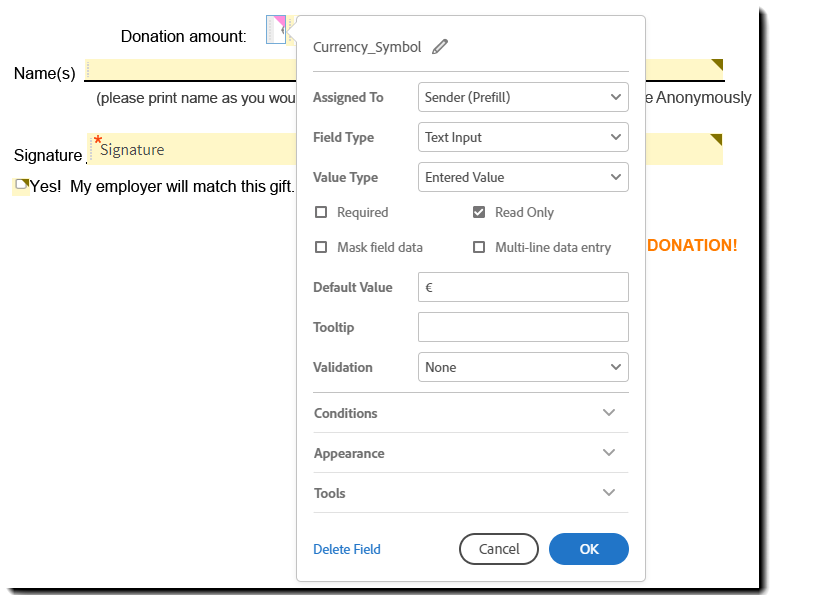Text source fields must be formatted as a Number (not Currency)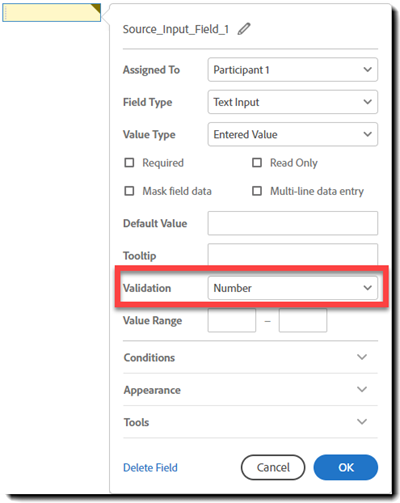Number validated source field

The field used to calculate the final value must be configured to have the:

• Display As value set to Number
• Number Format field set to the format you want to use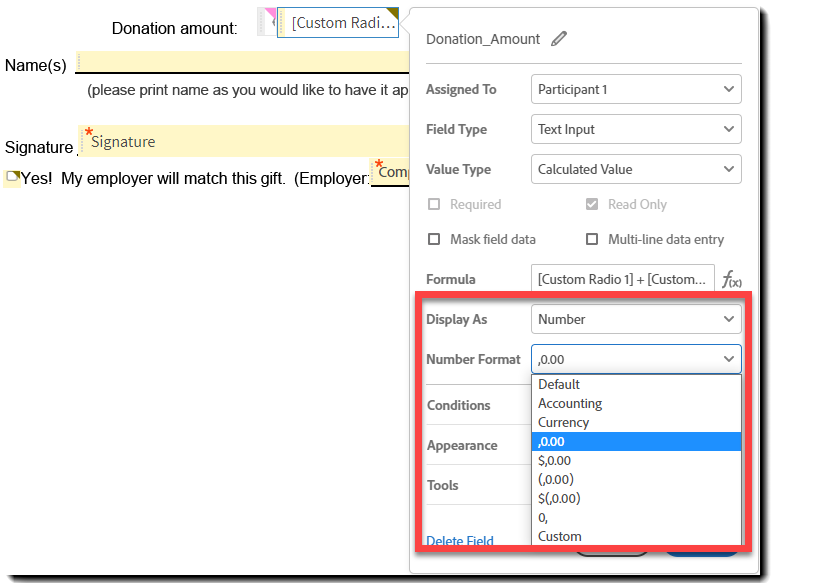## Calculated Fields Examples

• {{shipState_es_:signer1:calc([billingState]):showif(sameAs=unchecked)}}
• A field in the document assigned to the first signer that will only be displayed when another field (a check box) called “sameAs” is unchecked. The value of the field is automatically populated with the value of the field “billingState” that the signer has entered in the document.
• {{total_es_:signer1:calc(q1*pr1):format(Number,”\$,0.00”):align(right)}}
• A field in the document assigned to the first signer. Its value gets automatically calculated as the product of the values in fields “q1” and “pr1”. The resulting value is formatted as currency and displayed with a dollar (\$) symbol in front of the resulting value and containing two decimal points. The resulting value is also aligned right aligned within the field.
• price_es_:signer1:calc(if(pn1=”W1”,9.99,if(pn1=”W2”,5.99,if(pn1=”W3”,24.99,0))))}
• A field in the document called “price” which gets calculated based on the value selected by the signer in another fields “pn1” in the document. In this example if field “pn1” equals W1 the value displayed in the “price” field is 9.99, if field “pn1” equals W2 the value displayed in the “price” field is 5.99 and so on. If none of the values match the value of the field “pn1” the value displayed in “price” is 0. Using this mechanism, calculated fields can be used to automatically populate the price of an item based on the selection that a signer has made during signing.